# 1 三角函数

## 1.1 圆周率

\pi \approx 3.14159265f

## 1.2 余弦与正弦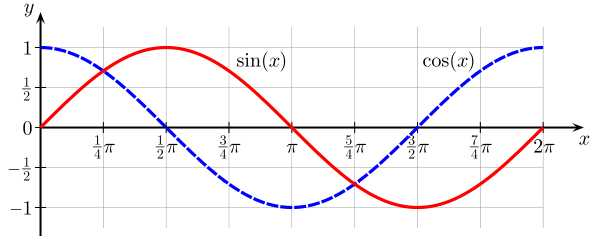## 1.3 单位圆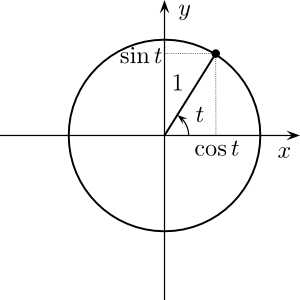t 是以弧度为单位的角度。

## 1.4 弧度与角度的转换

angleRad = angleDeg * Math.PI / 180;

angleDeg = angleRad * 180 / Math.PI;

# 2 向量

## 2.1 向量加法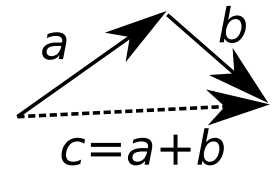a = c - b \\
b = c - a

## 2.2 单位向量 - 归一化向量

\hat{A} = \frac{\vec{A} }{\left \|\vec{A} \right \| }

## 2.3 向量点乘

\vec{A} \cdot \vec{B}=\sum_{i=1}^{n} A_{i} B_{i}=A_{1} B_{1}+A_{2} B_{2}+\cdots+A_{n} B_{n}

\theta = \arccos (\hat{A}\cdot\hat{B} ) \\
\theta = \arccos (\frac{\hat{A} \cdot \hat{B} }{\left \| \hat{A} \right \| \left \| \hat{B} \right \| } )

## 2.4 向量叉乘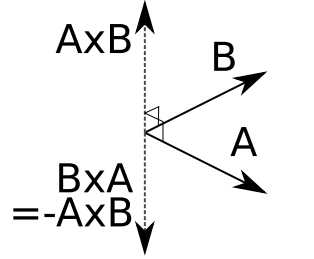\left[\begin{array}{l}
a_{1} \\
a_{2} \\
a_{3}
\end{array}\right] \times\left[\begin{array}{l}
b_{1} \\
b_{2} \\
b_{3}
\end{array}\right]=\left[\begin{array}{l}
a_{2} b_{3}-a_{3} b_{2} \\
a_{3} b_{1}-a_{1} b_{3} \\
a_{1} b_{2}-a_{2} b_{1}
\end{array}\right]

N = normalize(cross(A,B))

# 3 矩阵

## 3.1 单位矩阵

I_{3}=\left[\begin{array}{lll}
1 & 0 & 0 \\
0 & 1 & 0 \\
0 & 0 & 1
\end{array}\right]

## 3.2 矩阵与向量相乘

\left[\begin{array}{ccc}
a & b & c \\
d & e & f \\
g & h & i
\end{array}\right]\left[\begin{array}{l}
x \\
y \\
z
\end{array}\right]=\left[\begin{array}{c}
a x+b y+c z \\
d x+e y+f z \\
g x+h y+i z
\end{array}\right]

## 3.3 矩阵与矩阵相乘

A B=\left[\begin{array}{ll}
a & b \\
c & d
\end{array}\right]\left[\begin{array}{ll}
e & f \\
g & h
\end{array}\right]=\left[\begin{array}{ll}
a e+b g & a f+b h \\
c e+d g & c f+d h
\end{array}\right]

## 3.4 矩阵行列式

M=\left[\begin{array}{lll}
a & b & c \\
d & e & f \\
g & h & i
\end{array}\right]

|M|=a e i+b f g+c d h-c e g-b d i-a f h

## 3.5 矩阵转置

M=\left[\begin{array}{lll}
a & b & c \\
d & e & f \\
g & h & i
\end{array}\right]

M^{T}=\left[\begin{array}{lll}
a & d & g \\
b & e & h \\
c & f & i
\end{array}\right]

MM^{-1} = I

# 4 齐次矩阵

## 4.1 行主序齐次矩阵

v^{\prime}=\left[\begin{array}{llll}
V_{x} & V_{y} & V_{z} & 1
\end{array}\right]\left[\begin{array}{cccc}
X_{x} & X_{y} & X_{z} & 0 \\
Y_{x} & Y_{y} & Y_{z} & 0 \\
Z_{x} & Z_{y} & Z_{z} & 0 \\
T_{x} & T_{y} & T_{z} & 1
\end{array}\right]

## 4.2 列主序齐次矩阵

v^{\prime}=\left[\begin{array}{cccc}
X_{x} & Y_{x} & Z_{x} & T_{x} \\
X_{y} & Y_{y} & Z_{y} & T_{y} \\
X_{z} & Y_{z} & Z_{z} & T_{z} \\
0 & 0 & 0 & 1
\end{array}\right]\left[\begin{array}{c}
V_{x} \\
V_{y} \\
V_{z} \\
1
\end{array}\right]

## 4.3 平移、缩放、旋转

T=\left[\begin{array}{cccc}
1 & 0 & 0 & T_{x} \\
0 & 1 & 0 & T_{y} \\
0 & 0 & 1 & T_{z} \\
0 & 0 & 0 & 1
\end{array}\right]

S=\left[\begin{array}{cccc}
S_{x} & 0 & 0 & 0 \\
0 & S_{y} & 0 & 0 \\
0 & 0 & S_{z} & 0 \\
0 & 0 & 0 & 1
\end{array}\right]

R_{x}=\left[\begin{array}{cccc}
1 & 0 & 0 & 0 \\
0 & \cos (\theta) & -\sin (\theta) & 0 \\
0 & \sin (\theta) & \cos (\theta) & 0 \\
0 & 0 & 0 & 1
\end{array}\right]
R_{y}=\left[\begin{array}{cccc}
\cos (\theta) & 0 & \sin (\theta) & 0 \\
0 & 1 & 0 & 0 \\
-\sin (\theta) & 0 & \cos (\theta) & 0 \\
0 & 0 & 0 & 1
\end{array}\right]
R_{z}=\left[\begin{array}{cccc}
\cos (\theta) & -\sin (\theta) & 0 & 0 \\
\sin (\theta) & \cos (\theta) & 0 & 0 \\
0 & 0 & 1 & 0 \\
0 & 0 & 0 & 1
\end{array}\right]

## 4.4 视图矩阵

V=\left[\begin{array}{cccc}
R_{x} & R_{y} & R_{z} & -P_{x} \\
U_{x} & U_{y} & U_{z} & -P_{y} \\
-F_{x} & -F_{y} & -F_{z} & -P_{z} \\
0 & 0 & 0 & 1
\end{array}\right]

V=\left[\begin{array}{cccc}
1 & 0 & 0 & 0 \\
0 & 0 & -1 & 0 \\
0 & 1 & 0 & 0 \\
0 & 0 & 0 & 1
\end{array}\right]

## 4.5 投影矩阵

P=\left[\begin{array}{cccc}
S_{x} & 0 & 0 & 0 \\
0 & S_{y} & 0 & 0 \\
0 & 0 & S_{z} & P_{z} \\
0 & 0 & -1 & 0
\end{array}\right]

\begin{array}{l}
S_{x}=(2 * \text { near }) /(\text { range } * \text { aspect }+\text { range } * \text { aspect }) \\
S_{y}=\text { near/range } \\
S_{z}=-(\text { far }+\text { near }) /(\text { far }-\text { near }) \\
P_{z}=-(2 * \text { far } * \text { near }) /(\text { far }-\text { near }) \\
\text { range }=\tan (\text { fov } / 2) * \text { near }
\end{array}

## 4.6 常用的矩阵变换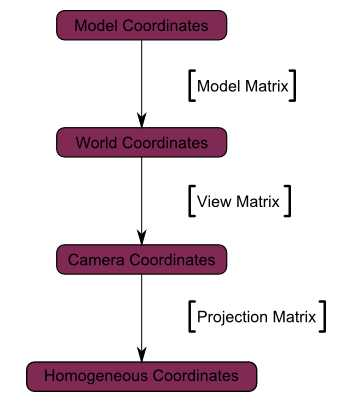Model Matrix：模型矩阵，即4.3节中所述的平移、缩放、旋转变换；
View Matrix：视图矩阵，即4.4节中所述的视图矩阵；
Projection Matrix：投影矩阵，即4.5节中所述的投影矩阵；